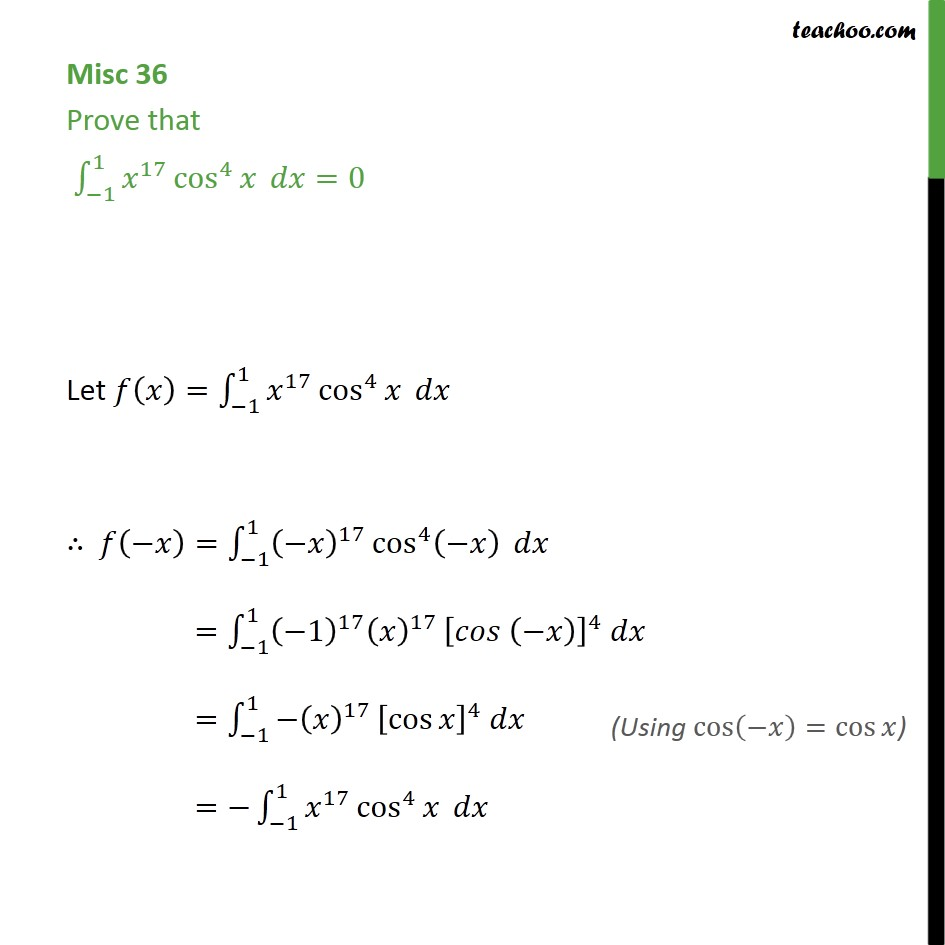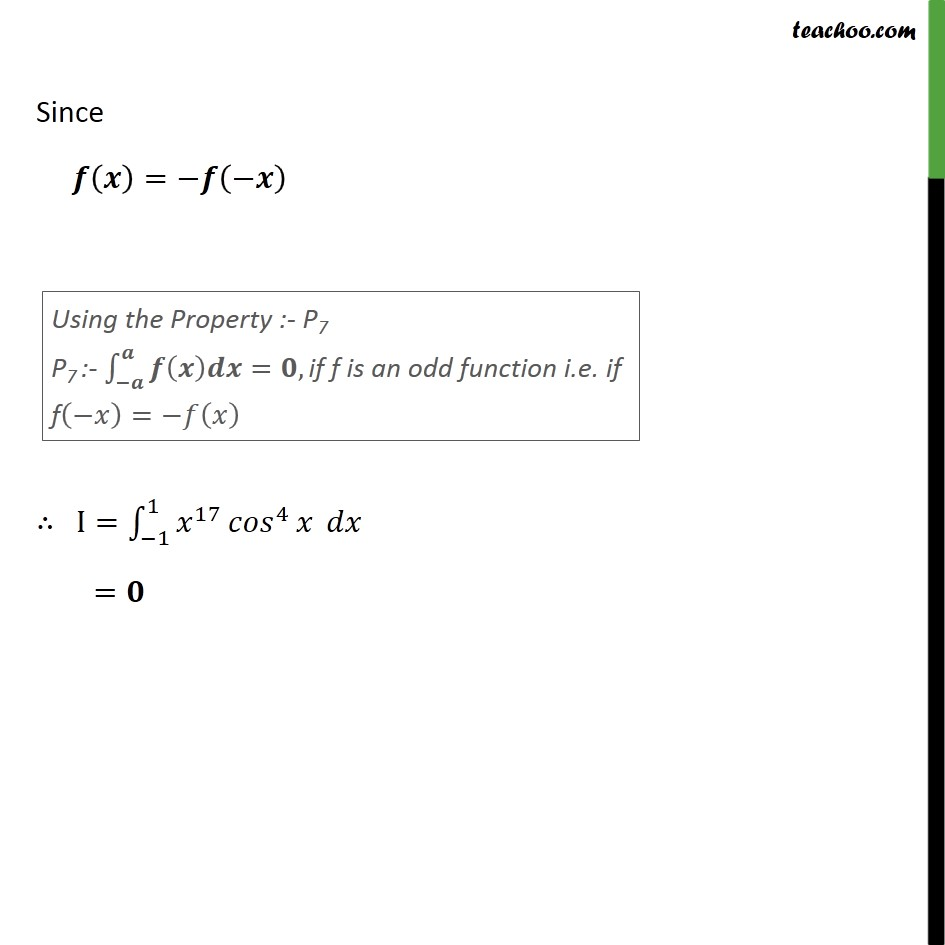Miscellaneous

Chapter 7 Class 12 Integrals
Serial order wiseLearn in your speed, with individual attention - Teachoo Maths 1-on-1 Class

### Transcript

Misc 34 Prove that 1 1 17 cos 4 =0 Let = 1 1 17 cos 4 = 1 1 17 cos 4 = 1 1 1 17 17 4 = 1 1 17 cos 4 = 1 1 17 cos 4 Since = I= 1 1 17 4 =